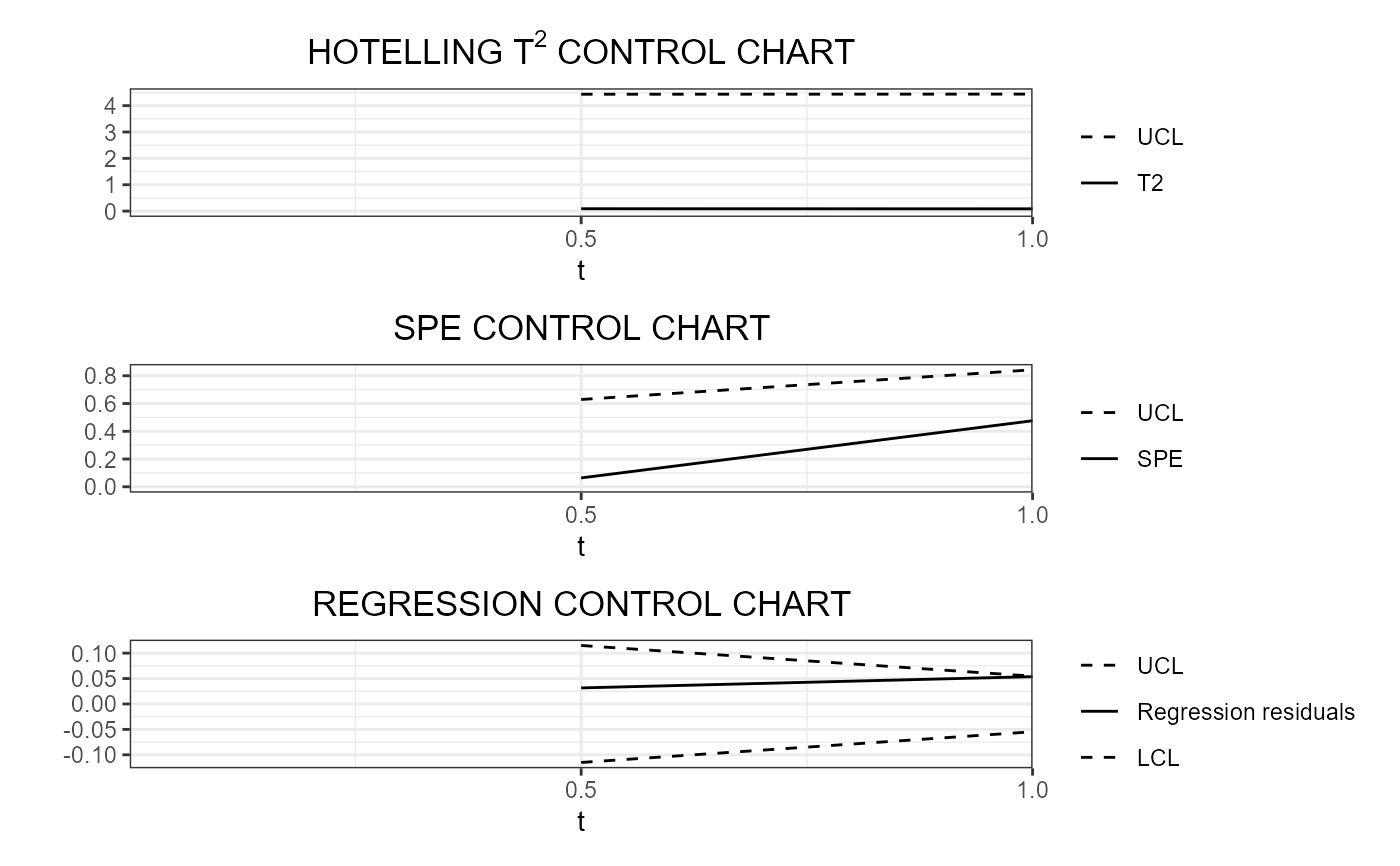This function produces a ggplot with the desired real-time control charts. It takes as input a list of data frames, produced with functions such as regr_cc_fof_real_time and control_charts_sof_pc_real_time, and the id of the observations for which real-time control charts are desired to be plotted. For each control chart, the solid line corresponds to the profile of the monitoring statistic and it is compared against control limits plotted as dashed lines. If a line is outside its limits it is coloured in red.

plot_control_charts_real_time(cclist, id_num)

## Arguments

cclist

A list of data frames, produced with functions such as regr_cc_fof_real_time and control_charts_sof_pc_real_time,

id_num

An index number giving the observation in the phase II data set to be plotted, i.e. 1 for the first observation, 2 for the second, and so on.

## Value

A ggplot with the real-time functional control charts.

## Details

If the line, representing the profile of the monitoring statistic over the functional domain, is out-of-control, then it is coloured in red.

regr_cc_fof_real_time, control_charts_sof_pc_real_time

## Examples

library(funcharts)
data("air")
air1 <- lapply(air, function(x) x[1:8, , drop = FALSE])
air2 <- lapply(air, function(x) x[9:10, , drop = FALSE])
mfdobj_x1_list <- get_mfd_list_real_time(air1[c("CO", "temperature")],
n_basis = 15,
lambda = 1e-2,
k_seq = c(0.5, 1))
mfdobj_x2_list <- get_mfd_list_real_time(air2[c("CO", "temperature")],
n_basis = 15,
lambda = 1e-2,
k_seq = c(0.5, 1))
y1 <- rowMeans(air1$NO2) y2 <- rowMeans(air2$NO2)
mod_list <- sof_pc_real_time(y1, mfdobj_x1_list)
cclist <- regr_cc_sof_real_time(
mod_list = mod_list,
y_new = y2,
mfdobj_x_new = mfdobj_x2_list,
include_covariates = TRUE)
plot_control_charts_real_time(cclist, 1)